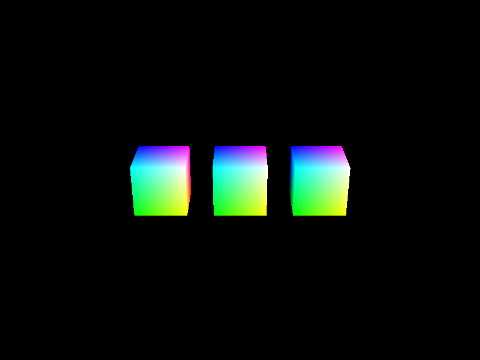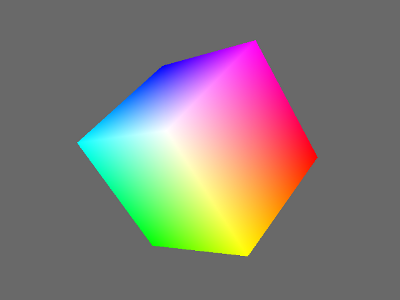# Cg Tutorial Part 1

## Cg Tutorial Part 1: The Baseline Panda App

We’ll start by setting up the tutorial project folder. Create a new folder for the tutorial and download the tutorial media then put the files in the new folder. The zip file contains all the models and textures you will need to follow this tutorial series.

For this first lesson we’ll just create a basic Panda python script. We will be modifying this python script as we continue the other lessons. Copy the script below and save it to the folder.

```# Lesson1.py
import sys

import direct.directbase.DirectStart
from direct.interval.LerpInterval import LerpFunc
from panda3d.core import Texture, TextureStage

base.setBackgroundColor(0.0, 0.0, 0.0)
base.disableMouse()

base.camLens.setNearFar(1.0, 50.0)
base.camLens.setFov(45.0)

camera.setPos(0.0, -20.0, 10.0)
camera.lookAt(0.0, 0.0, 0.0)

root = render.attachNewNode("Root")
root.setPos(0.0, 0.0, 0.0)

textureArrow.setWrapU(Texture.WMClamp)
textureArrow.setWrapV(Texture.WMClamp)

stageArrow = TextureStage("Arrow")
stageArrow.setSort(1)

textureCircle.setWrapU(Texture.WMClamp)
textureCircle.setWrapV(Texture.WMClamp)

stageCircle = TextureStage("Circle")
stageCircle.setSort(2)

cubes = []
for x in [-3.0, 0.0, 3.0]:
cube = modelCube.copyTo(root)
cube.setPos(x, 0.0, 0.0)
cubes += [ cube ]

base.accept("escape", sys.exit)

base.accept("o", base.oobe)

def animate(t):
for i in range(len(cubes)):
cubes[i].setH(t * (2.0 ** i))

interval = LerpFunc(animate, 5.0, 0.0, 360.0)

base.accept("i", interval.start)

def move(x, y, z):
root.setX(root.getX() + x)
root.setY(root.getY() + y)
root.setZ(root.getZ() + z)

base.accept("d", move, [1.0, 0.0, 0.0])
base.accept("a", move, [-1.0, 0.0, 0.0])
base.accept("w", move, [0.0, 1.0, 0.0])
base.accept("s", move, [0.0, -1.0, 0.0])
base.accept("e", move, [0.0, 0.0, 1.0])
base.accept("q", move, [0.0, 0.0, -1.0])

base.run()
```

If you run that script, you’ll get the following output below. The controls are q, w, e, a, s, d for moving the camera; ‘o’ for moving the camera via the mouse, ‘i’ to start the cubes rotating and ‘esc’ to quit. You will be modifying this script as you follow this tutorial series.## 3D Models, Shaders and Hardware

Now that we have the basic script, lets take a look at how 3d models, shaders and the 3d hardware interact with each other. Please open the file cube.egg with a text editor. Egg files are human readable. We will need this information later on to understand how the vertex shader and the fragment shader works. You can also see how the model looks like in panda by using the pview model viewer.```// A vertex entry in an egg file
<Group> {
<VertexPool> Cube {
<Vertex> 0 {
1.0 1.0 -1.0
<UV> { 1.0 1.0 }
RGBA> { 1.0 0.0 0.0 1.0 }
}
...
```

The cube has six faces. Each face has four different vertices. Therefore this cube has 24 vertices. Theoretically a cube only needs eight vertices with each vertex being shared by three faces. The problem with this is that each vertex can only have one color, but what happens if we want each of the six faces to be a different color? This is impossible if the cube is only defined with eight vertices. There are more disadvantages if we only define the cube with eight vertices, which we will talk about later on. The only advantage of having less vertices is that we have to send less vertices to the graphic card but in almost all applications vertices are not a limiting factor. The memory consumption of vertices in comparison to the memory consumption of textures is negligible. Besides the color entry for a vertex, a vertex also has one UV entry associated with it.

Next look at the colors defined in the egg file. If you compare all the color entries, you will only find eight unique colors in the egg file. Why does the model have thousands of colors when viewed in the model viewer then? This is because of linear interpolation, where a value is generated between two different values based on a “distance”. Today graphic cards are very good at linear interpolation with the ability to do billions of linear interpolations per second. The downside is that sometimes the graphic card can ONLY do linear interpolation and you can’t change that, even with a shader.

Back to the colors. If you have a red color (1.0, 0.0, 0.0) on one vertex and a dark blue color (0.0, 0.0, 0.5) on the other vertex the graphic card simply interpolates the color for every pixel between this two vertices, even without shaders (only if requested, but Panda3D ask the graphic card to do this). The graphic card doesn’t know that a color comes in three parts: Red, Green and Blue. It only knows that it is manipulating values, in this case adjusting the constituent values for Red Green and Blue. Here is an example of how the graphic card interpolates:

Red Vertex

Blue Vertex

Color value

100%

0%

(1.0, 0.0, 0.0)

75%

25%

(0.75, 0.0, 0.125)

50%

50%

(0.5, 0.0, 0.25)

25%

75%

(0.25, 0.0, 0.375)

0%

100%

(0.0, 0.0, 0.5)

A simplified version of how the graphic card draws the model (in reality it does not work exactly like this but the result is the same): If the graphic card needs to draw a pixel on a screen it first looks if this pixel is on a vertex. If it is, it can take the color of the vertex and draw a pixel with this color. If not, the graphic card looks at which triangle this pixel belongs. Then it looks at where the vertices of this triangle are and calculates the distance to each of the vertices. Based on this distance and the color of the vertices, it interpolates all color components and draws a pixel with this color.

A blur filter (like in the glow example) is an example of such a setup. You need at least two passes to create such an effect.

## Modifying the Script

We will now modify the script to see how the normal 3D pipeline blends the vertex colors with textures. In the tutorial media, there are two textures, ‘arrow.png’ and ‘circle.png’. We will apply these to the cubes using only Panda.

Place one of the following lines in the script after the cubes are placed in the scenegraph:

```root.setTexture(stageArrow, textureArrow)
root.setTexture(stageCircle, textureCircle)
```

You will notice that the textures get applied to all of the cubes. Now try placing the textures on individual cubes:

```cubes.setTexture(stageArrow, textureArrow)
cubes.setTexture(stageCircle, textureCircle)
cubes.setTexture(stageArrow, textureArrow)
cubes.setTexture(stageCircle, textureCircle)
```

Now that we have a general idea of how 3D hardware and models work, lets move on to using shaders.

Part 2: The simplest possible shader